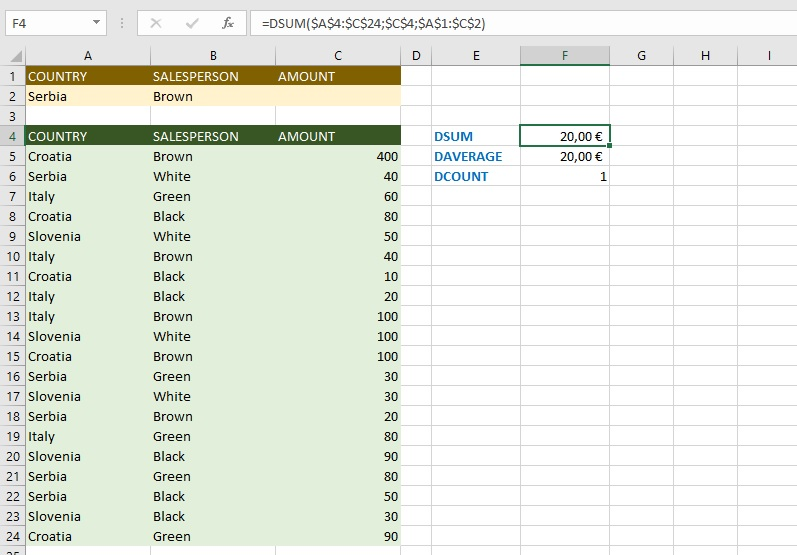# “D” functions

The “D” functions are used for multiple data aggregation in the given table. In essence, they do the same thing as “IFS” functions in a slightly more elegant way, and whether this way is better – you estimate yourself! By the way, I am an adversary when the “experts” describe tables as databases. Sometimes the database actually makes one table, but this is very rare. Now, at least you know what letter “D” in ther prefix stands for!

In this “recipe” it will be about how DSUM, DAVERAGE, and DCOUNT work. The syntax of all these functions is essentially the same as the DSUM:

DSUM (<database>,<field>,<criteria>)

The first argument (database) is the range of cells or the name of a structured table. The second (field) is the name or regular column number that contains the values over which aggregation is performed, in the case of DSUM it is addition! The third argument (criteria) is the range or table that contains the criteria for performing the aggregation.

For example, if in the given table (A4: C24) we want to collect the values (C4 or the regular column number, here it would be number 3) with the application of the given criteria (A1: C2) the formula reads:

=DSUM(\$A\$4:\$C\$24;\$C\$4;\$A\$1:\$C\$2)

If the country of Serbia is listed in the table of criteria and the salesperson is Brown then the sum is 20 €. Similarly, by using DAVERAGE we can calculate the average amount, and using DCOUNT to count the number of occurrences of such transactions.